# NCERT Solutions, Determinants, Part -2, Class 12, Maths Notes - Class 12

## Class 12: NCERT Solutions, Determinants, Part -2, Class 12, Maths Notes - Class 12

The document NCERT Solutions, Determinants, Part -2, Class 12, Maths Notes - Class 12 is a part of Class 12 category.
All you need of Class 12 at this link: Class 12

Determinants

Exercise 4.2

Question 1: Using the property of determinants and without expanding, prove that: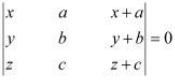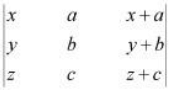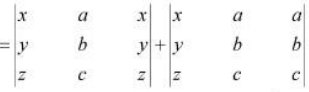= 0+0 = 0

Question 2: Using the property of determinants and without expanding, prove that: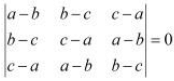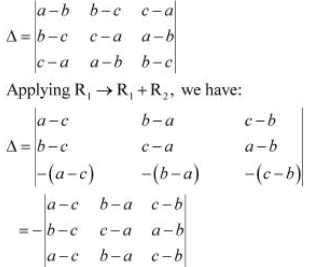Here, the two rows R1 and R3 are identical.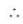Δ = 0.

Question 3: Using the property of determinants and without expanding, prove that: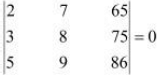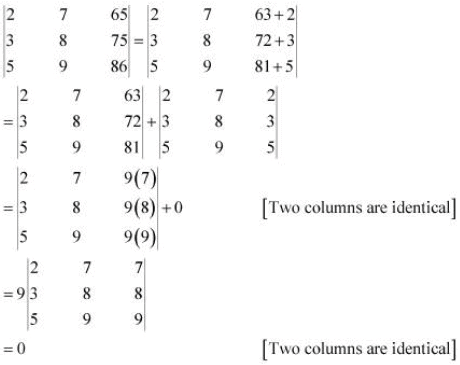Question 4: Using the property of determinants and without expanding, prove that: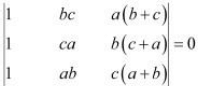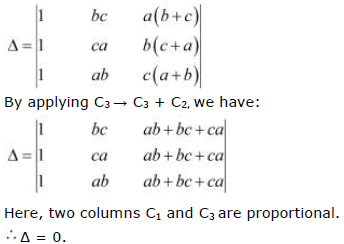Question 5:
Using the property of determinants and without expanding, prove that: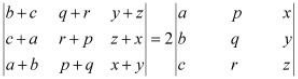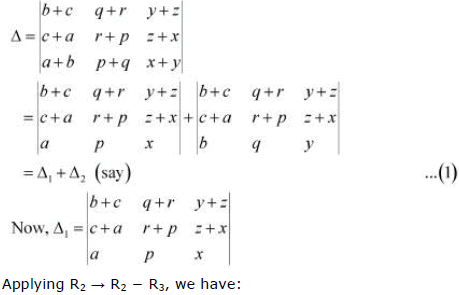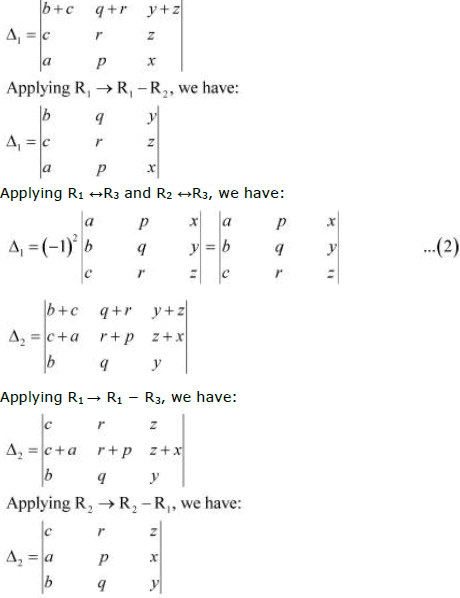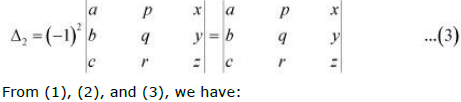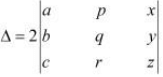Hence, the given result is proved.

Question 6: By using properties of determinants, show that: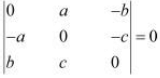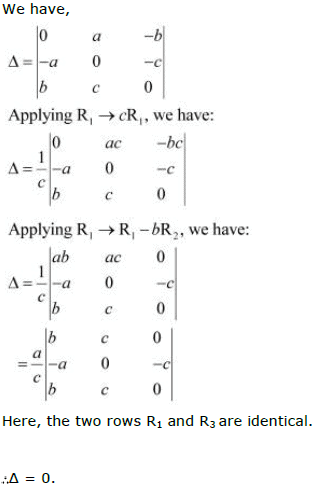Question 7: By using properties of determinants, show that: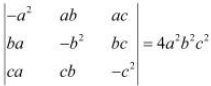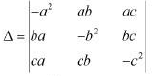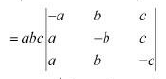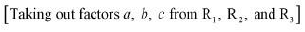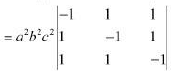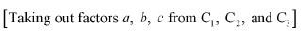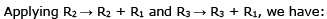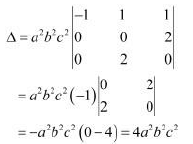Question 8: By using properties of determinants, show that: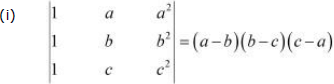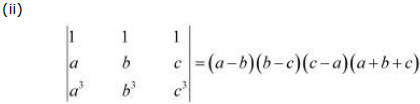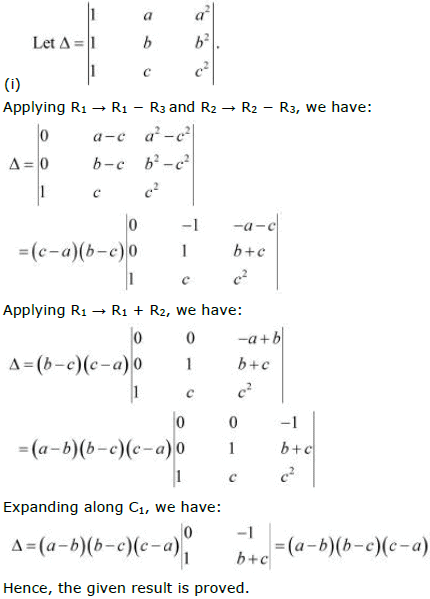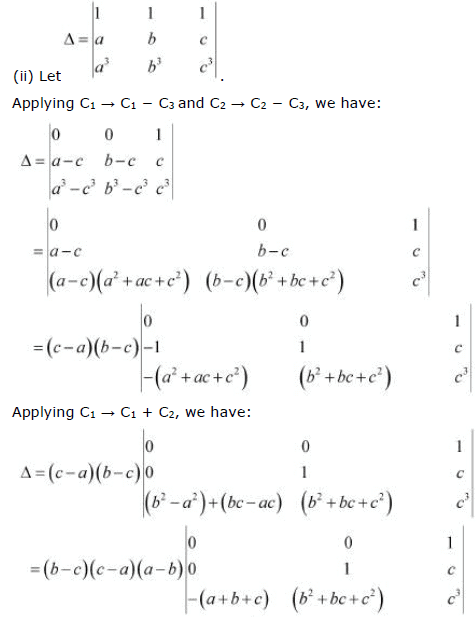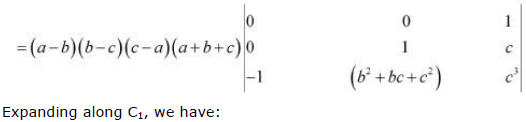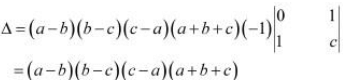Hence, the given result is proved.

Question 9: By using properties of determinants, show that: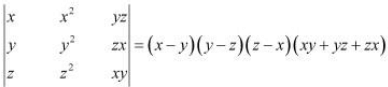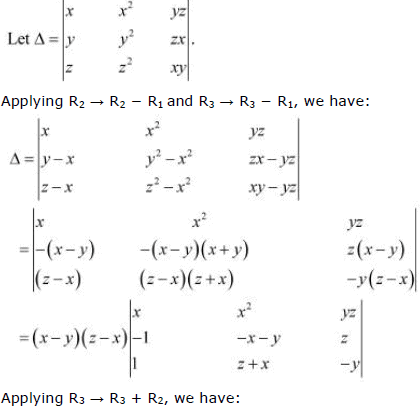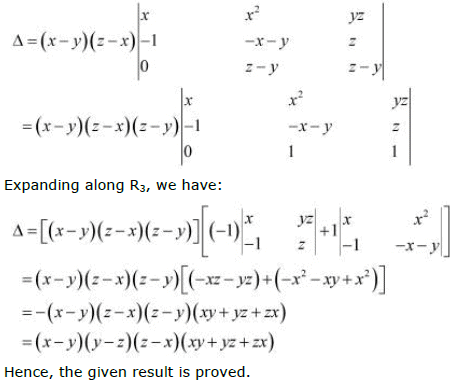Question 10: By using properties of determinants, show that: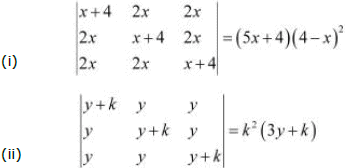(i)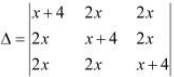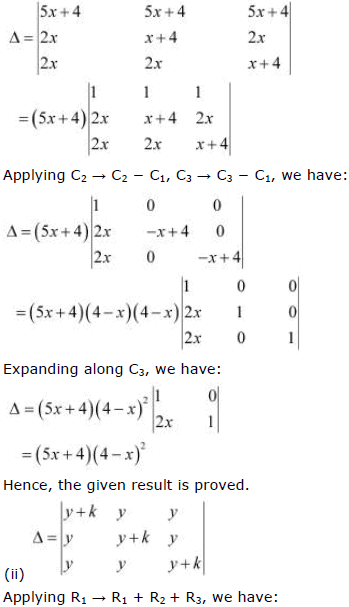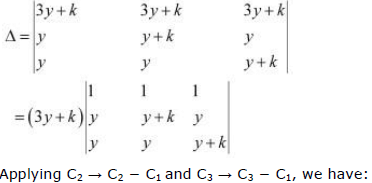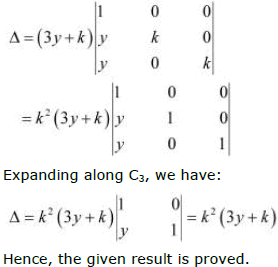Question 11: By using properties of determinants, show that: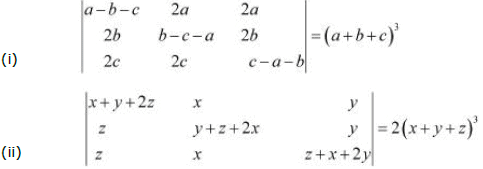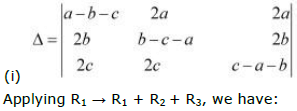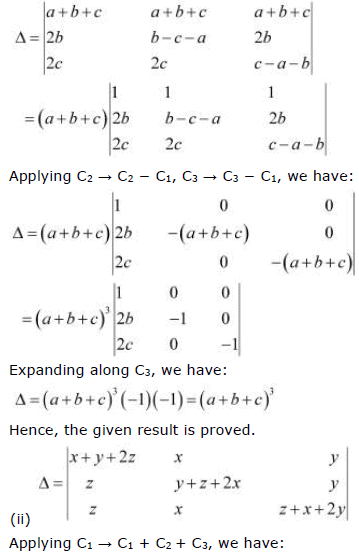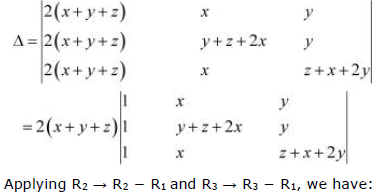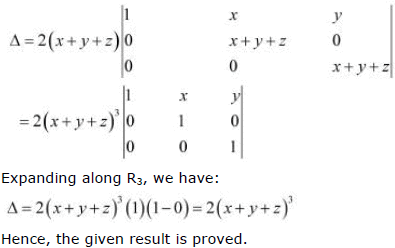Question 12: By using properties of determinants, show that: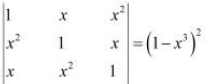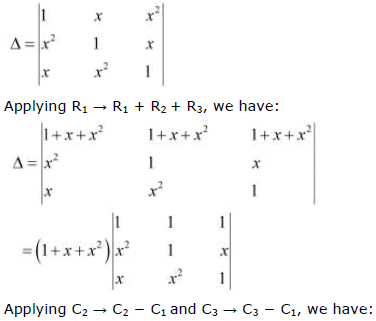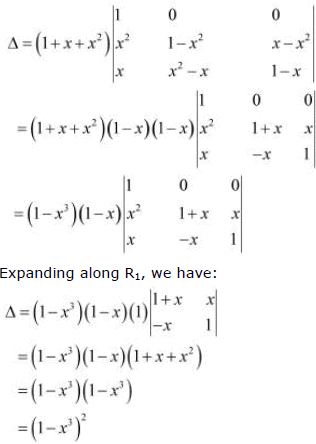Hence, the given result is proved.

Question 13: By using properties of determinants, show that: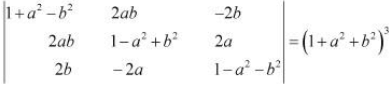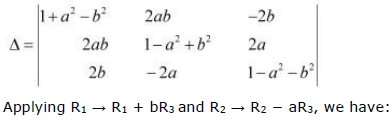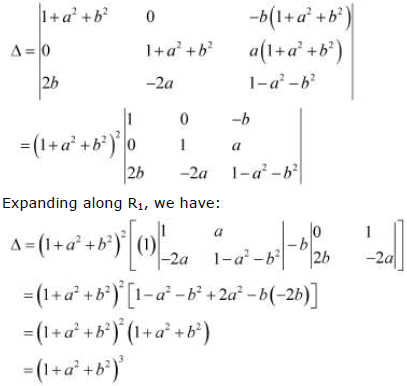Question 14:
By using properties of determinants, show that: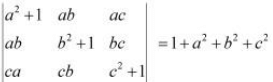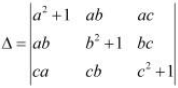Taking out common factors a, b, and c from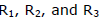respectively, we have: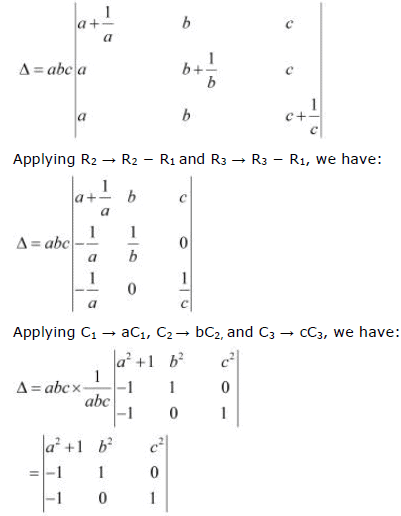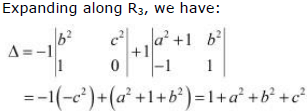Hence, the given result is proved.

Question 15: Choose the correct answer.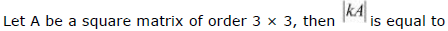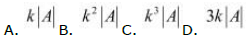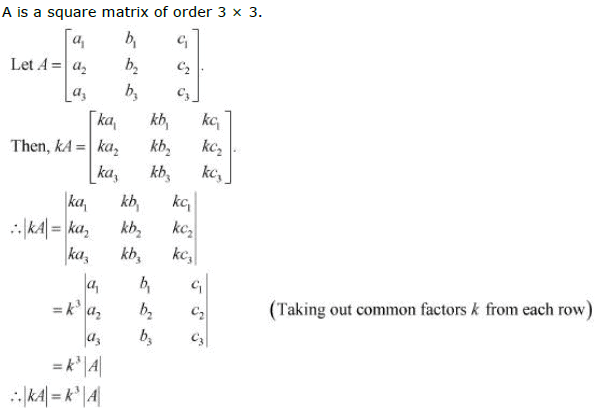Hence, the correct answer is C.

Question 16: Which of the following is correct?
A. Determinant is a square matrix.
B. Determinant is a number associated to a matrix.
C. Determinant is a number associated to a square matrix.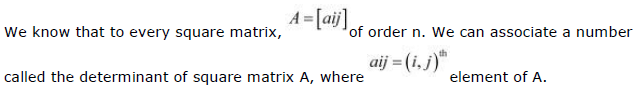Thus, the determinant is a number associated to a square matrix.
Hence, the correct answer is C.

The document NCERT Solutions, Determinants, Part -2, Class 12, Maths Notes - Class 12 is a part of Class 12 category.
All you need of Class 12 at this link: Class 12Use Code STAYHOME200 and get INR 200 additional OFF

Track your progress, build streaks, highlight & save important lessons and more!

,

,

,

,

,

,

,

,

,

,

,

,

,

,

,

,

,

,

,

,

,

,

,

,

,

,

,

,

,

,

,

,

,

;